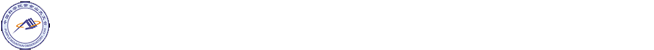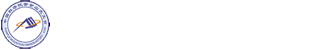Skip to contentAn Accurate P3M Algorithm for Gravitational Lensing Studies in Simulations

Title: An Accurate P3M Algorithm for Gravitational Lensing Studies in Simulations

Speaker: Mr. Kun Xu (Shanghai Jiao Tong University)

Time: 14:00pm, Apr. 02, 2021

Location: 5-516, PMO Xianlin Campus

Abstract: We present a two-dimensional (2D) Particle-Particle-Particle-Mesh (P3M) algorithm with an optimized Green function and adaptive softening length for gravitational lensing studies in N-Body simulations. The analytical form of the optimized Green function G(k) is given. The softening schemes (S) are studied for both the PM and the PP calculations in order for accurate force calculation and suppression of the particle discreteness effect. Our method is two orders of magnitude more accurate than the simple PM algorithm with the poor man’s Green function (∝ 1/k2 ) at a scale of a few mesh cells or smaller. The force anisotropy is also much smaller than the conventional PM calculation. We can achieve a force accuracy better than 0.1 percent at all scales with our algorithm. When we apply the algorithm to computing lensing quantities in N-Body simulations, the errors are dominated by the Poisson noise caused by particle discreteness. The Poisson noise can be suppressed by smoothing out the particle distribution, which can be achieved by simply choosing an adaptive softening length in the PP calculation. We have presented a criterion to set the adaptive softening length. Our algorithm is also applicable to cosmological simulations. We provide a python implementation P3M lens for this algorithm.